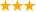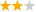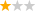# 利物浦大学金融数学专业

## 01利物浦大学金融数学专业解析

• 所属国家： 英国
• 大学： 利物浦大学
• 课程学制： 1年
• 开学时间： 9月
• 用户口碑： (0)条口碑
• 就业率： N/A
• 专业排名： N/A
• 学费： 20350.00英镑/年

## 02利物浦大学金融数学专业介绍

This MSc Financial Mathematics programme will equip you with the mathematical and computational skills required in the finance and insurance industries. - Probability theory, stochastic analysis and mathematical modelling - Computational methods - Financial derivatives - Risk management - Econometrics This program is ideal for professionals with mathematical background who would like to start, or further develop a career in the finance and insurance industries. It is also an excellent training ground for students wishing to embark upon a research degree (PhD) in financial or actuarial mathematics.

## 03利物浦大学金融数学专业世界排名

 2022 2021 2020 2019 2018 2016 2017 2015 2014 2013 180+ 180+ 180+ 160+ 170+ 150+ N/A N/A N/A N/A

## 04利物浦大学金融数学专业课程

1.选修课:

2.必修课:

 Compulsory modules Probability Essentials for Financial Calculus (MATH480) Interest Rate Theory (MATH481) Stochastic Modelling In Finance (MATH482) Stochastic Analysis and Its Applications (MATH483) Computational Methods In Financial Mathematics (MATH484) Econometric and Statistical Methods (ECON814) Corporate Finance I (ECON906) Main Dissertation (MATH554) 必修课程 金融微积分概率必需品(MATH480) 利率理论(MATH481) 随机建模在金融(MATH482) 随机分析及其应用(MATH483) 金融数学计算方法(MATH484) 计量经济学和统计学方法(ECON814) 我公司财务(ECON906) 主要论文(MATH554) Optional modules Financial Engineering (ECON918) Advanced Econometrics (ECON920) 选修课程 金融工程(ECON918) 高级计量经济学(ECON920)

## 提交评估，获取录取几率和2022年招生手册

•好评中评差评